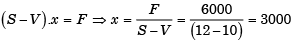Courses

# Line Balancing & Break Even Analysis - MCQ Test 1

## 20 Questions MCQ Test RRB JE for Mechanical Engineering | Line Balancing & Break Even Analysis - MCQ Test 1

Description
This mock test of Line Balancing & Break Even Analysis - MCQ Test 1 for Mechanical Engineering helps you for every Mechanical Engineering entrance exam. This contains 20 Multiple Choice Questions for Mechanical Engineering Line Balancing & Break Even Analysis - MCQ Test 1 (mcq) to study with solutions a complete question bank. The solved questions answers in this Line Balancing & Break Even Analysis - MCQ Test 1 quiz give you a good mix of easy questions and tough questions. Mechanical Engineering students definitely take this Line Balancing & Break Even Analysis - MCQ Test 1 exercise for a better result in the exam. You can find other Line Balancing & Break Even Analysis - MCQ Test 1 extra questions, long questions & short questions for Mechanical Engineering on EduRev as well by searching above.
QUESTION: 1

### The table given details of an assembly line.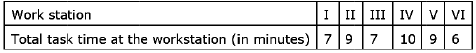What is the line efficiency of the assembly line ?

Solution: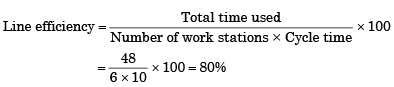QUESTION: 2

### A standard machine tool and an automatic machine tool are being compared for the production of a component. Following data refers to the two machines.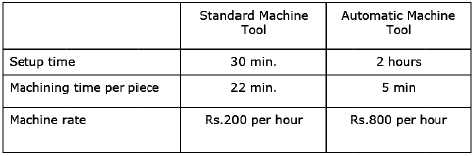The breakeven production batch size above which the automatic machine tool will be economical to use, will be

Solution: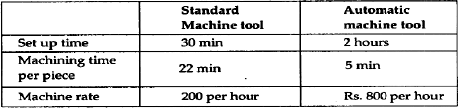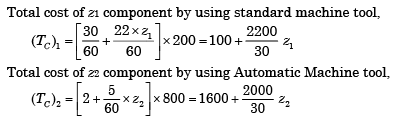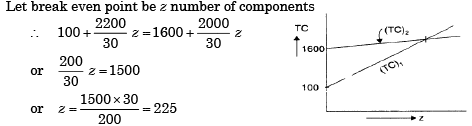Alternately
Let N be the Break even number
At Break even Point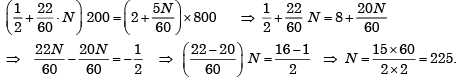QUESTION: 3

### In an assembly line for assembling toys, five workers are assigned tasks which take times of 10, 8, 6, 9 and 10 minutes respectively. The balance delay for the line is:

Solution:

Balance delay = 100 – Line efficiency (in percentage).

QUESTION: 4

A company produces two types of toys: P and Q. Production time of Q is twice that of P and the company has a maximum of 2000 time units per day. The supply of raw material is just sufficient to produce 1500 toys (of any type) per day. Toy type Q requires an electric switch which is available @ 600 pieces per day only. The company makes a profit of Rs. 3 and Rs. 5 on type P and Q respectively. For maximization of profits, the daily production quantities of P and Q toys should respectively be:

Solution:

Clearly,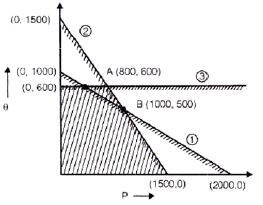QUESTION: 5

An electronic equipment manufacturer has decided to add a component sub-assembly operation that can produce 80 units during a regular 8-hour shift. This operation consists of three activities as below: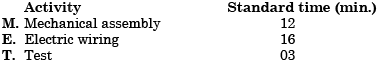For line balancing the number of work stations required for the activities M, E and T would respectively be

Solution:
QUESTION: 6

A component can be produced by any of the four processes I, II, III and IV. Process I has a fixed cost of Rs. 20 and variable cost of Rs. 3 per piece. Process II has a fixed cost Rs. 50 and variable cost of Re. 1 per piece. Process III has a fixed cost of Rs. 40 and variable cost of Rs. 2 per piece. Process IV has a fixed cost of Rs. 10 and variable cost of Rs. 4 per piece. If the company wishes to produce 100 pieces of the component, from economic point of view it should choose

Solution: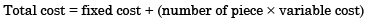QUESTION: 7

The product structure of an assembly P is shown in the figure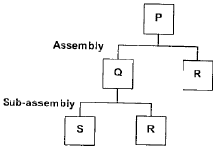Estimated demand for end product P is as follows: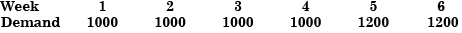Ignore lead times for assembly and sub-assembly. Production capacity (per week) for component R is the bottleneck operation. Starting with zero inventory, the smallest capacity that will ensure a feasible production plan up to week 6 is:

Solution:
QUESTION: 8

Two machines of the same production rate are available for use. On machine 1, the fixed cost is Rs. 100 and the variable cost is Rs. 2 per piece produced. The corresponding numbers for the machine 2 are Rs. 200 and Re. 1 respectively. For certain strategic reasons both the machines are to be used concurrently. The sale price of the first 300 units is Rs. 3.50 per unit and subsequently it is only Rs. 3.00. The breakeven production rate for each machine is:

Solution:
QUESTION: 9

Consider the following chara cteristics of assembly line balancing:

1. shareout of sequential work activities into work stations
2. High utilization of equipment
3. Minimization of idle time

Which of the statements given above are correct?

Solution:

All the three statements are correct with respect to assembly line balancing:
1. Apportionment of sequential work activities into work stations
2. High utilization of equipment
3. Minimization of idle time

QUESTION: 10

Last year, a manufacturer produced 15000 products which were sold for Rs. 300 each. At that volume, the fixed costs were Rs. 15.2 lacs and total variable costs were Rs. 21 lacs. The break even quantity of product would be:

Solution: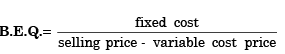QUESTION: 11

Which of the following are the benefits of assembly line balancing?

1. It minimises the in-process inventory
2. It reduces the work content.
3. It smoothens the production flow
4. It maintains the required rate of output.

Select the correct answer using the codes given below:

Solution:
QUESTION: 12

Assertion (A): It is possible to have more than one break-even point in break even charts.

Reason (R): All variable costs are directly variable with production.

Solution:
QUESTION: 13

In an assembly line, what is the balance delay?

Solution:
QUESTION: 14

On a lathe, the actual machining time required per work piece is 30 minutes. Two types of carbide tools are available, both having a tool life of 60 minutes.

Type I : Brazed type of original cost Rs. 50/-.
Type II : Throwaway tip (square) of original cost Rs. 70/-
If the overall cost of grinding the cutting edge is Rs. 10/-, assuming all the costs are the same for both the types, for break even costs, the appropriate batch size would be:

Solution:
QUESTION: 15

In an assembly line, when the workstation times are unequal, the overall production rate of an assembly line is determined by the:

Solution:
QUESTION: 16

Two alternative methods can produce a product first method has a fixed cost of Rs. 2000/- and variable cost of Rs. 20/- per piece. The second method has a fixed cost of Rs. 1500/- and a variable cost of Rs. 30/-. The break even quantity between the two alternatives is:

Solution:

2000 + 20n = 1500 + 30n,  10n = 500 and n = 50.

QUESTION: 17

Which one of the following is true in respect of production control for continuous or assembly line production?

Solution:
QUESTION: 18

For a small scale industry, the fixed cost per month is Rs. 5000/-. The variable cost per product is Rs. 20/- and sales price is Rs. 30/- per piece. The break-even production per month will be:

Solution:

Break even production per month is 500.

QUESTION: 19

Manufacturing a product requires processing on four machines A, B, C, D in the order A – B – C – D. The capacities of four machines are A = 100, B = 110, C = 120 and D = 130 units per shift. If the expected output is 90% of the system capacity, then what is the expected output?

Solution:

In the sequence of A – B – C – D only minimum output have to be calculated. Other machines will be on empty position.
∴ Output = η × 100= 0.9 × 100= 90 units

QUESTION: 20

In the production of a product the fixed costs are Rs. 6,000/- and the variable cost is Rs. 10/- per product. If the sale price of the product is Rs. 12/-, the break even volume of products to be made will be:

Solution: